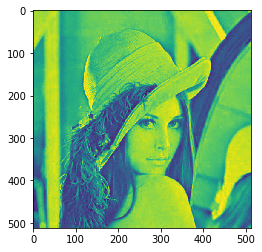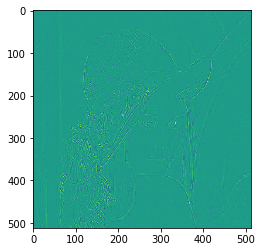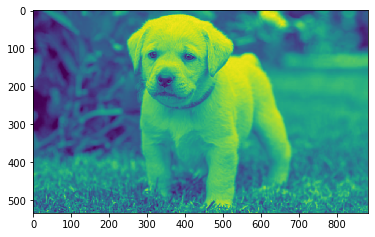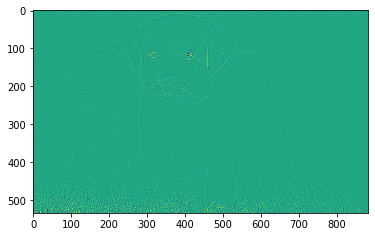GFG App
Open AppBrowser
Continue

# Mahotas – 2D Laplacian filter

In this article we will see how we can apply 2D laplacian filter to the image in mahotas. A Laplacian filter is an edge detector used to compute the second derivatives of an image, measuring the rate at which the first derivatives change. This determines if a change in adjacent pixel values is from an edge or continuous progression.

In this tutorial we will use “lena” image, below is the command to load it.

`mahotas.demos.load('lena')`

Below is the lena imageIn order to do this we will use mahotas.laplacian_2D method
Syntax : mahotas.laplacian_2D(img)
Argument : It takes image object as argument
Return : It returns image object

Note : Input image should be filtered or should be loaded as grey

In order to filter the image we will take the image object which is numpy.ndarray and filter it with the help of indexing, below is the command to do this

`image = image[:, :, 0]`

Below is the implementation

## Python3

 `# importing required libraries` `import` `mahotas` `import` `mahotas.demos` `from` `pylab ``import` `gray, imshow, show` `import` `numpy as np` `import` `matplotlib.pyplot as plt` `  `  `# loading image` `img ``=` `mahotas.demos.load(``'lena'``)`     `  `  `# filtering image` `img ``=` `img.``max``(``2``)`   `print``(``"Image"``)` `  `  `# showing image` `imshow(img)` `show()`   `# applying 2D Laplacian filter` `new_img ``=` `mahotas.laplacian_2D(img)` ` `    `# showing image` `print``(``"2D Laplacian filter"``)` `imshow(new_img)` `show()`

Output :

`Image`2D Laplacian filterAnother example

## Python3

 `# importing required libraries` `import` `mahotas` `import` `numpy as np` `from` `pylab ``import` `gray, imshow, show` `import` `os` `import` `matplotlib.pyplot as plt` ` `  `# loading image` `img ``=` `mahotas.imread(``'dog_image.png'``)`     `# filtering image` `img ``=` `img[:, :, ``0``]` `  `  `print``(``"Image"``)` `  `  `# showing image` `imshow(img)` `show()`   `# applying 2D Laplacian filter` `new_img ``=` `mahotas.laplacian_2D(img)` ` `    `# showing image` `print``(``"2D Laplacian filter"``)` `imshow(new_img)` `show()`

Output :

`Image`2D Laplacian filterMy Personal Notes arrow_drop_up